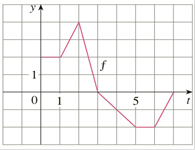Chapter 4.3, Problem 3E

Chapter
Section
Textbook Problem

# Let g ( x ) = ∫ 0 x f ( t ) d t , where f is the function whose graph is shown.(a) Evaluate g ( 0 ) , g ( 1 ) , g ( 2 ) , g ( 3 ) , and g ( 6 ) .(b) On what interval is g increasing?(c) Where does g have a maximum value?(d) Sketch a rough graph of g.To determine

a)

To evaluate:

i. g0

ii. g1

iii. g2

iv. g3

v. g6

Explanation

Given:

gx=0xf(t)dt

Calculation:

i. To evaluate g0

The integral of a function over a trivial interval is zero so

g0=00f(t)dt =0

ii. To evaluate g1

g1=01f(t)dt

Geometrically g(1)  is the area under the graph from 0 to 1.

The area is two whole squares

g1=2

iii. To evaluate g2

The area is four whole squares and triangle with base 1 and height 2

g2=02f(t)dt=01f(t)dt+12f(t)dt

g2=2+2+12·2·1=5

iv

To determine

b)

To find:

On what interval is g increasing

To determine

c)

To find:

Where does  g have maximum value

To determine

d)

To sketch:

A rough graph of g

### Still sussing out bartleby?

Check out a sample textbook solution.

See a sample solution

#### The Solution to Your Study Problems

Bartleby provides explanations to thousands of textbook problems written by our experts, many with advanced degrees!

Get Started

#### Find the median for the following set of scores: 1, 9, 3, 6, 4, 3, 11, 10

Essentials of Statistics for The Behavioral Sciences (MindTap Course List)

#### In Exercises 107-120, factor each expression completely. 120. x3 27

Applied Calculus for the Managerial, Life, and Social Sciences: A Brief Approach

#### Solve

Mathematical Applications for the Management, Life, and Social Sciences

#### Evaluate the integral. 38. /4/2csc4cot4d

Single Variable Calculus: Early Transcendentals

#### The quadratic approximation for at a = 1 is:

Study Guide for Stewart's Single Variable Calculus: Early Transcendentals, 8th

#### True or False: converges if and only if .

Study Guide for Stewart's Multivariable Calculus, 8th